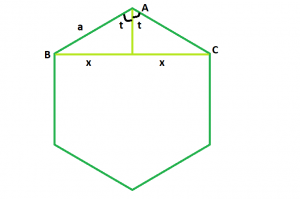# Length of Diagonal of a n-sided regular polygon

Given a n-sided regular polygon of side length a.The task is to find the length of it’s diagonal.

Examples:

```Input:  a = 9, n = 10
Output:  17.119

Input: a = 4, n = 5
Output: 6.47213
```## Recommended: Please try your approach on {IDE} first, before moving on to the solution.

Approach:

We know that the sum of interior angles of a polygon = (n – 2) * 180 where, n is the no. of sides in the polygon.
So, each interior angle = (n – 2) * 180/n
Now, we have to find BC = 2 * x. If we draw a perpendicular AO on BC, we will see that the perpendicular bisects BC in BO and OC, as triangles AOB and AOC are congruent to each other.
Now, t = (n – 2) * 180/2n
So, sint = x/a
Therefore, x = asint
Hence, diagonal=2x = 2asint = 2asin((n – 2) * 180/2n)

## C++

 `// C++ Program to find the diagonal ` `// of a regular polygon with given side length ` `#include ` `using` `namespace` `std; ` ` `  `// Function to find the diagonal ` `// of a regular polygon ` `float` `polydiagonal(``float` `n, ``float` `a) ` `{ ` ` `  `    ``// Side and side length cannot be negative ` `    ``if` `(a < 0 && n < 0) ` `        ``return` `-1; ` ` `  `    ``// diagonal ` `    ``// degree converted to radians ` `    ``return` `2 * a * ``sin``((((n - 2) * 180) / (2 * n)) * 3.14159 / 180); ` `} ` ` `  `// Driver code ` `int` `main() ` `{ ` `    ``float` `a = 9, n = 10; ` `    ``cout << polydiagonal(n, a) << endl; ` ` `  `    ``return` `0; ` `} `

## Java

 `// Java Program to find the diagonal ` `// of a regular polygon with given side length ` ` `  `class` `GFG { ` ` `  `// Function to find the diagonal ` `// of a regular polygon ` `    ``static` `float` `polydiagonal(``float` `n, ``float` `a) { ` ` `  `        ``// Side and side length cannot be negative ` `        ``if` `(a < ``0` `&& n < ``0``) { ` `            ``return` `-``1``; ` `        ``} ` ` `  `        ``// diagonal ` `        ``// degree converted to radians ` `        ``return` `(``float``) (``2` `* a * Math.sin((((n - ``2``) * ``180``) / (``2` `* n)) * ``3.14159` `/ ``180``)); ` `    ``} ` ` `  `// Driver code ` `    ``public` `static` `void` `main(String[] args) { ` `        ``float` `a = ``9``, n = ``10``; ` `        ``System.out.printf(``"%.3f"``,polydiagonal(n, a)); ` ` `  `    ``} ` `}  ` ` `  `// This code is contributed by 29AjayKumar `

## Python3

 `# Python3 Program to find the diagonal  ` `# of a regular polygon with given side length  ` `import` `math as mt ` ` `  `# Function to find the diagonal  ` `# of a regular polygon ` `def` `polydiagonal(n, a):  ` ` `  `    ``# Side and side length cannot  ` `    ``# be negative  ` `    ``if` `(a < ``0` `and` `n < ``0``):  ` `        ``return` `-``1` ` `  `    ``# diagonal degree converted to radians  ` `    ``return` `(``2` `*` `a ``*` `mt.sin((((n ``-` `2``) ``*` `180``) ``/`  `           ``(``2` `*` `n)) ``*` `3.14159` `/` `180``))  ` ` `  `# Driver code  ` `a, n ``=` `9``, ``10` `print``(polydiagonal(n, a)) ` ` `  `# This code is contributed ` `# by Mohit kumar 29 `

## C#

 `// C#  Program to find the diagonal ` `// of a regular polygon with given side length ` `using` `System; ` ` `  `public` `class` `GFG{ ` `     `  `// Function to find the diagonal ` `// of a regular polygon ` `    ``static` `float` `polydiagonal(``float` `n, ``float` `a) { ` ` `  `        ``// Side and side length cannot be negative ` `        ``if` `(a < 0 && n < 0) { ` `            ``return` `-1; ` `        ``} ` ` `  `        ``// diagonal ` `        ``// degree converted to radians ` `        ``return` `(``float``) (2 * a * Math.Sin((((n - 2) * 180) / (2 * n)) * 3.14159 / 180)); ` `    ``} ` ` `  `// Driver code ` `    ``static` `public` `void` `Main (){ ` `            ``float` `a = 9, n = 10; ` `        ``Console.WriteLine(polydiagonal(n, a)); ` ` `  `    ``} ` `}  ` ` `  `// This code is contributed by  @Sachin... `

## PHP

 ` `

Output:

```17.119
```

Attention reader! Don’t stop learning now. Get hold of all the important DSA concepts with the DSA Self Paced Course at a student-friendly price and become industry ready.

My Personal Notes arrow_drop_upBudding Web DeveloperKeen learnerAverage CoderDancer&Social Activist

If you like GeeksforGeeks and would like to contribute, you can also write an article using contribute.geeksforgeeks.org or mail your article to contribute@geeksforgeeks.org. See your article appearing on the GeeksforGeeks main page and help other Geeks.

Please Improve this article if you find anything incorrect by clicking on the "Improve Article" button below.